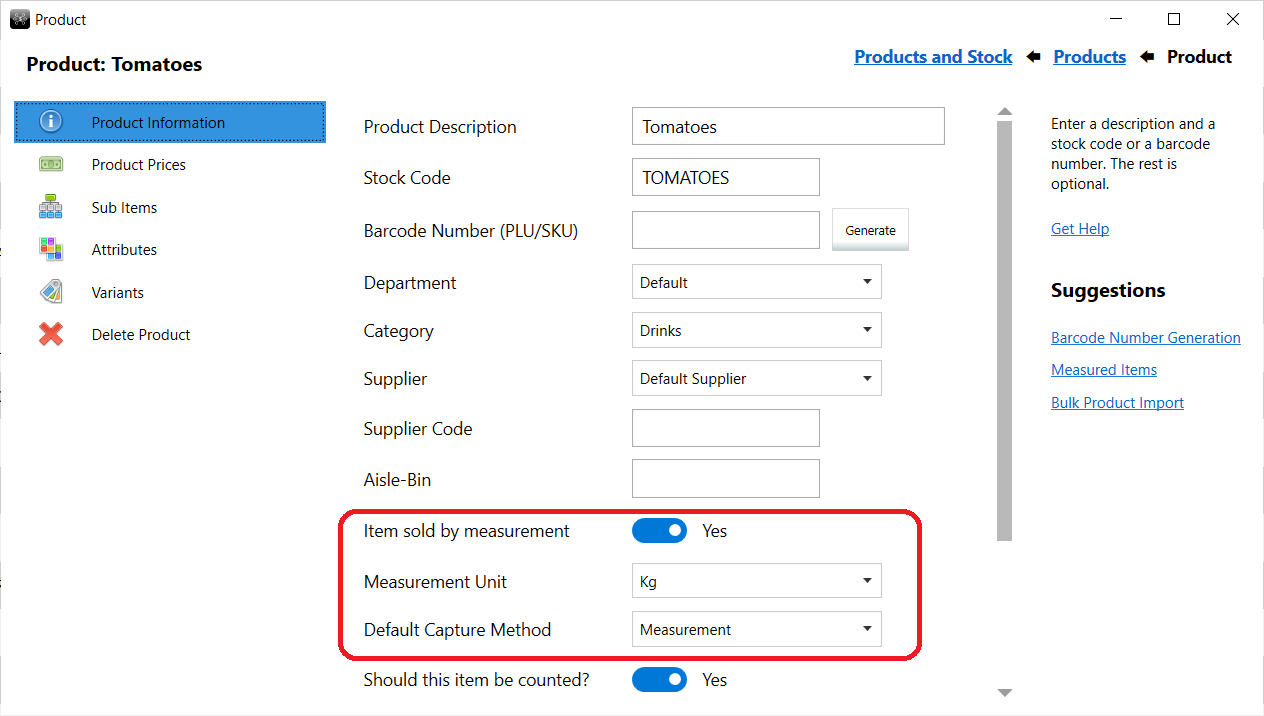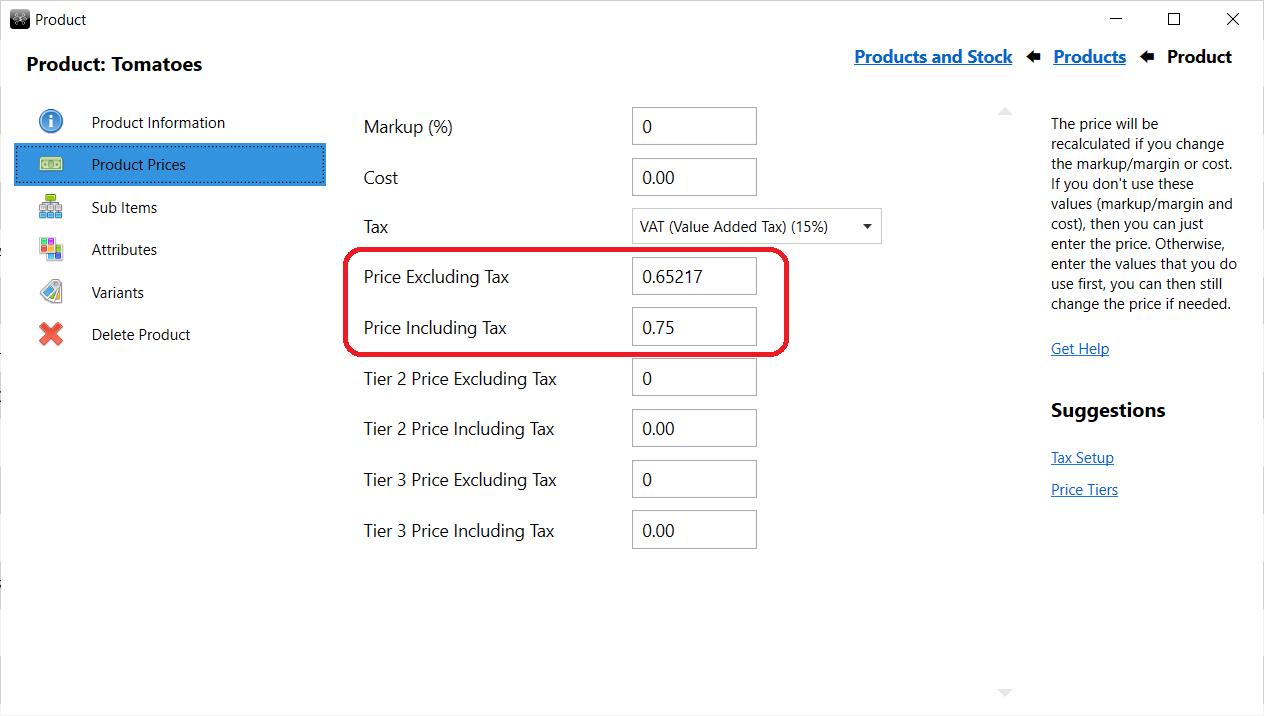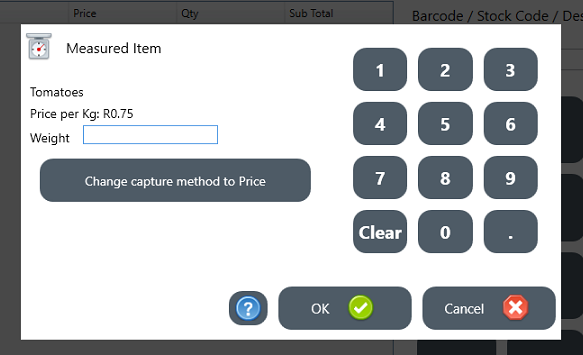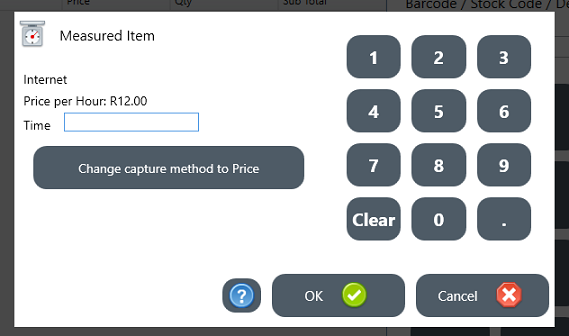ROBOTILL HELP

## Measured Items and Scale Items

Note: You use this setup if your scale does not print barcodes with the price and/or weight in the barcode. If it does, see Scale Generated Barcodes

Measured Items are any item that are measured in some way (weight, length, time, etc).

For example, you can create a product 'Tomatoes' that will be weighed.Select that the item is sold by measurement. You can choose the measurement item (for example kilograms or grams - you can add or edit measurement items if needed). You can also choose the default capture method but it can be changed at the till if needed. You can for example enter the weight and then the price will be calculated - or you can enter the price then the quantity (weight) will be calculated.The price you enter will be the price per unit. In this example the price per Kg.

#### At the till

If the item is scanned or if the cashier type in Tomatoes (or select it), the following screen will appear:The cashier can then just enter the weight and the system will calculate the price. The cashier can also change the default capture method and enter the price instead of the weight. In that case the weight (quantity) will be calculated.

Another example of a measured item would be a shop that sell Internet time on a computer. You can set up a Internet stock item and choose the unit as time.
When that item is selected, the cashier will be prompted to enter the time and the amount will be calculated.© ROBOTILL (PTY) LTD - www.robotill.com